# Class IsoInterval<T extends Temporal<? super T>,​I extends IsoInterval<T,​I>>

java.lang.Object
net.time4j.range.IsoInterval<T,​I>
Type Parameters:
`T` - temporal type of time points within a given interval
`I` - generic self-referencing interval type
All Implemented Interfaces:
`ChronoInterval<T>`
Direct Known Subclasses:
`ClockInterval`, `DateInterval`, `MomentInterval`, `TimestampInterval`

public abstract class IsoInterval<T extends Temporal<? super T>,​I extends IsoInterval<T,​I>> extends Object implements ChronoInterval<T>

Represents an abstract temporal interval on a timeline for ISO-8601-types.

Note that the start of an interval is (almost) always included (with the exception of intervals with infinite past). The end is open for intervals with infinite future and else included for date intervals by default and excluded for other interval types. This default setting can be overwritten however (although potentially harmful for the performance).

Since:
2.0
Author:
Meno Hochschild
• ## Method Summary

Modifier and Type
Method
Description
`boolean`
`abuts​(ChronoInterval<T> other)`
Queries if this interval abuts the other one such that there is neither any overlap nor any gap between.
`I`
`collapse()`
Changes this interval to an empty interval with the same start anchor.
`boolean`
`contains​(ChronoInterval<T> other)`
Does this interval contain the other one?
`boolean`
`contains​(T temporal)`
Queries if given time point belongs to this interval.
`boolean`
`enclosedBy​(I other)`
ALLEN-relation: Equivalent to `other.encloses(this)`.
`boolean`
`encloses​(I other)`
ALLEN-relation: Does this interval enclose the other one such that this start is before the start of the other one and this end is after the end of the other one?
`boolean`
`equals​(Object obj)`
Compares the boundaries (start and end) and also the time axis of this and the other interval.
`boolean`
`equivalentTo​(I other)`
ALLEN-relation: Does this interval equal the other one taking into account the open or closed state of the boundaries?
`Optional<I>`
`findIntersection​(ChronoInterval<T> other)`
Obtains the intersection of this interval and other one if present.
`boolean`
`finishedBy​(I other)`
ALLEN-relation: Equivalent to `other.finishes(this)`.
`boolean`
`finishes​(I other)`
ALLEN-relation: Does this interval finish the other one such that both end time points are equal and the start of this interval is after the start of the other one?
`<R> R`
`get​(ChronoFunction<ChronoInterval<T>,​R> function)`
Lets given query evaluate this interval.
`Boundary<T>`
`getEnd()`
Yields the upper bound of this interval.
`Boundary<T>`
`getStart()`
Yields the lower bound of this interval.
`int`
`hashCode()`

`boolean`
`intersects​(ChronoInterval<T> other)`
Queries if this interval intersects the other one such that there is at least one common time point.
`boolean`
`isAfter​(ChronoInterval<T> other)`
Is this interval after the other one?
`boolean`
`isAfter​(T temporal)`
Is this interval after the given time point?
`boolean`
`isBefore​(ChronoInterval<T> other)`
Is this interval before the other one?
`boolean`
`isBefore​(T temporal)`
Is this interval before the given time point?
`boolean`
`isEmpty()`
Determines if this interval is empty.
`boolean`
`meets​(I other)`
ALLEN-relation: Does this interval precede the other one such that there is no gap between?
`boolean`
`metBy​(I other)`
ALLEN-relation: Equivalent to `other.meets(this)`.
`boolean`
`overlappedBy​(I other)`
ALLEN-relation: Equivalent to `other.overlaps(this)`.
`boolean`
`overlaps​(I other)`
ALLEN-relation: Does this interval overlaps the other one such that the start of this interval is still before the start of the other one?
`boolean`
`precededBy​(I other)`
ALLEN-relation: Equivalent to `other.precedes(this)`.
`boolean`
`precedes​(I other)`
ALLEN-relation: Does this interval precede the other one such that there is a gap between?
`String`
`print​(ChronoPrinter<T> printer)`
Prints the canonical form of this interval using a localized interval pattern.
`void`
```print​(ChronoPrinter<T> startFormat, char separator, ChronoPrinter<T> endFormat, BracketPolicy policy, InfinityStyle infinityStyle, Appendable buffer)```
Prints this interval in a technical format using given formatters and separator.
`String`
```print​(ChronoPrinter<T> printer, String intervalPattern)```
Prints the canonical form of this interval in a custom format.
`String`
```print​(ChronoPrinter<T> printer, BracketPolicy policy)```
Prints the start and end separated by a slash using given formatter (technical format).
`boolean`
`startedBy​(I other)`
ALLEN-relation: Equivalent to `other.starts(this)`.
`boolean`
`starts​(I other)`
ALLEN-relation: Does this interval start the other one such that both start time points are equal and the end of this interval is before the end of the other one?
`I`
`toCanonical()`
Changes this interval to an interval such that calendrical intervals become closed intervals and other intervals become half-open.
`String`
`toString()`
Yields a descriptive string using the standard output of the method `toString()` of start and end.
`I`
`withClosedEnd()`
Includes the upper boundary of this interval.
`I`
`withClosedStart()`
Includes the lower boundary of this interval.
`I`
`withEnd​(UnaryOperator<T> operator)`
Yields a copy of this interval with given operator applied on end time.
`I`
`withEnd​(T temporal)`
Yields a copy of this interval with given end time.
`I`
`withOpenEnd()`
Excludes the upper boundary from this interval.
`I`
`withOpenStart()`
Excludes the lower boundary from this interval.
`I`
`withStart​(UnaryOperator<T> operator)`
Yields a copy of this interval with given operator applied on start time.
`I`
`withStart​(T temporal)`
Yields a copy of this interval with given start time.
`<V> ValueInterval<T,​I,​V>`
`withValue​(V value)`
Creates a combination of this interval with an associated value.

### Methods inherited from class java.lang.Object

`getClass, notify, notifyAll, wait, wait, wait`

### Methods inherited from interface net.time4j.range.ChronoInterval

`isFinite`
• ## Method Details

• ### getStart

public final  getStart()
Description copied from interface: `ChronoInterval`

Yields the lower bound of this interval.

Specified by:
`getStart` in interface `ChronoInterval<T extends Temporal<? super T>>`
Returns:
start interval boundary
• ### getEnd

public final  getEnd()
Description copied from interface: `ChronoInterval`

Yields the upper bound of this interval.

Specified by:
`getEnd` in interface `ChronoInterval<T extends Temporal<? super T>>`
Returns:
end interval boundary
• ### get

public final <R> R get(ChronoFunction<ChronoInterval<T>,​R> function)

Lets given query evaluate this interval.

Type Parameters:
`R` - generic type of result of query
Parameters:
`function` - interval query
Returns:
result of query or `null` if undefined
Throws:
`ChronoException` - if the given query is not executable
Since:
4.24
`HolidayModel.firstBusinessDay()`, `HolidayModel.lastBusinessDay()`
• ### withStart

public I withStart(T temporal)

Yields a copy of this interval with given start time.

Parameters:
`temporal` - new start timepoint
Returns:
changed copy of this interval
Throws:
`IllegalArgumentException` - if new start is after end
Since:
2.0
• ### withEnd

public I withEnd(T temporal)

Yields a copy of this interval with given end time.

Parameters:
`temporal` - new end timepoint
Returns:
changed copy of this interval
Throws:
`IllegalArgumentException` - if new end is before start
Since:
2.0
• ### withStart

public I withStart(UnaryOperator<T> operator)

Yields a copy of this interval with given operator applied on start time.

Parameters:
`operator` - operator to be applied on the start
Returns:
changed copy of this interval
Throws:
`IllegalStateException` - if the start boundary is infinite
`IllegalArgumentException` - if new start is after end
Since:
4.18
`withEnd(UnaryOperator)`
• ### withEnd

public I withEnd(UnaryOperator<T> operator)

Yields a copy of this interval with given operator applied on end time.

Example:

```     PlainDate start = PlainDate.of(2014, 2, 27);
PlainDate end = PlainDate.of(2014, 5, 20);
DateInterval interval = DateInterval.between(start, end).withEnd(PlainDate.DAY_OF_MONTH.maximized());
System.out.println(interval); // [2014-02-27/2014-05-31]
```
Parameters:
`operator` - operator to be applied on the end
Returns:
changed copy of this interval
Throws:
`IllegalStateException` - if the end boundary is infinite
`IllegalArgumentException` - if new end is before start
Since:
4.18
`withStart(UnaryOperator)`
• ### withOpenStart

public I withOpenStart()

Excludes the lower boundary from this interval.

Returns:
changed copy of this interval excluding lower boundary
Since:
4.21
• ### withClosedStart

public I withClosedStart()

Includes the lower boundary of this interval.

Returns:
changed copy of this interval including lower boundary
Throws:
`IllegalStateException` - if the start is infinite past
Since:
4.21
• ### withOpenEnd

public I withOpenEnd()

Excludes the upper boundary from this interval.

Returns:
changed copy of this interval excluding upper boundary
Since:
2.0
• ### withClosedEnd

public I withClosedEnd()

Includes the upper boundary of this interval.

Returns:
changed copy of this interval including upper boundary
Throws:
`IllegalStateException` - if the end is infinite future
Since:
2.0
• ### isEmpty

public boolean isEmpty()
Description copied from interface: `ChronoInterval`

Determines if this interval is empty.

Specified by:
`isEmpty` in interface `ChronoInterval<T extends Temporal<? super T>>`
Returns:
`true` if this interval does not contain any time point else `false`
• ### isBefore

public boolean isBefore(T temporal)
Description copied from interface: `ChronoInterval`

Is this interval before the given time point?

Specified by:
`isBefore` in interface `ChronoInterval<T extends Temporal<? super T>>`
Parameters:
`temporal` - reference time point
Returns:
`true` if this interval is before given time point else `false`
• ### isBefore

public boolean isBefore(ChronoInterval<T> other)

Is this interval before the other one?

Equivalent to the expression `(precedes(other) || meets(other))`.

Specified by:
`isBefore` in interface `ChronoInterval<T extends Temporal<? super T>>`
Parameters:
`other` - another interval whose relation to this interval is to be investigated
Returns:
`true` if this interval is before the other one else `false`
Since:
2.0
• ### isAfter

public boolean isAfter(T temporal)
Description copied from interface: `ChronoInterval`

Is this interval after the given time point?

Specified by:
`isAfter` in interface `ChronoInterval<T extends Temporal<? super T>>`
Parameters:
`temporal` - reference time point
Returns:
`true` if this interval is after given time point else `false`
• ### isAfter

public boolean isAfter(ChronoInterval<T> other)

Is this interval after the other one?

Equivalent to the expression `(precededBy(other) || metBy(other))`.

Specified by:
`isAfter` in interface `ChronoInterval<T extends Temporal<? super T>>`
Parameters:
`other` - another interval whose relation to this interval is to be investigated
Returns:
`true` if this interval is after the other one else `false`
Since:
2.0
• ### contains

public boolean contains(T temporal)
Description copied from interface: `ChronoInterval`

Queries if given time point belongs to this interval.

Specified by:
`contains` in interface `ChronoInterval<T extends Temporal<? super T>>`
Parameters:
`temporal` - time point to be queried
Returns:
`true` if given time point belongs to this interval else `false`
• ### contains

public boolean contains(ChronoInterval<T> other)

Does this interval contain the other one?

In contrast to `encloses(I)` the interval boundaries may also be equal.

Specified by:
`contains` in interface `ChronoInterval<T extends Temporal<? super T>>`
Parameters:
`other` - another interval whose relation to this interval is to be investigated
Returns:
`true` if this interval contains the other one else `false`
Since:
2.0
`ChronoInterval.intersects(ChronoInterval)`
• ### collapse

public I collapse()

Changes this interval to an empty interval with the same start anchor.

Returns:
new empty interval with same start (anchor always inclusive)
Throws:
`IllegalStateException` - if the start is infinite
Since:
2.0
• ### toCanonical

public I toCanonical()

Changes this interval to an interval such that calendrical intervals become closed intervals and other intervals become half-open.

The temporal space will not be changed. Infinite boundaries also remain unchanged.

Returns:
new interval with canonical boundaries
Throws:
`IllegalStateException` - if there is no canonical form (for example for [00:00/24:00])
Since:
3.9/4.6
• ### equals

public final boolean equals(Object obj)

Compares the boundaries (start and end) and also the time axis of this and the other interval.

Note: Two intervals which are `equivalentTo(I)` to each other are not always equal to each other. For example a half-open date interval whose end is one day later than the end of a closed date interval with same start is considered equivalent, but not equal.

Overrides:
`equals` in class `Object`
Parameters:
`obj` - object to be compared with this
Returns:
`true` if given object is also an interval on the same time axis and has the same boundaries as this interval else `false`
• ### hashCode

public final int hashCode()
Overrides:
`hashCode` in class `Object`
• ### toString

public final String toString()

Yields a descriptive string using the standard output of the method `toString()` of start and end.

Overrides:
`toString` in class `Object`
Returns:
String
• ### print

public String print(ChronoPrinter<T> printer)

Prints the canonical form of this interval using a localized interval pattern.

If given printer does not contain a reference to a locale then the interval pattern "{0}/{1}" will be used. Note: Starting with version v2.0 and before v3.9/4.6, this method had a different behaviour and just delegated to `print(printer, BracketPolicy.SHOW_WHEN_NON_STANDARD)`.

Parameters:
`printer` - format object for printing start and end
Returns:
localized formatted string
Throws:
`IllegalStateException` - if the canonicalization of this interval fails
`IllegalArgumentException` - if an interval boundary is not formattable
Since:
3.9/4.6
`toCanonical()`, `print(ChronoPrinter, String)`, `FormatPatternProvider.getIntervalPattern(Locale)`
• ### print

public String print(ChronoPrinter<T> printer, String intervalPattern)

Prints the canonical form of this interval in a custom format.

Example:

```  DateInterval interval = DateInterval.since(PlainDate.of(2015, 1, 1));
ChronoFormatter<PlainDate> formatter =
ChronoFormatter.ofDatePattern("MMM d, uuuu", PatternType.CLDR, Locale.US);
System.out.println(interval.print(formatter, "since {0}"));
// output: since Jan 1, 2015
```
Parameters:
`printer` - format object for printing start and end components
`intervalPattern` - interval pattern containing placeholders {0} and {1} (for start and end)
Returns:
formatted string in given pattern format
Throws:
`IllegalStateException` - if the canonicalization of this interval fails
`IllegalArgumentException` - if an interval boundary is not formattable
Since:
3.9/4.6
`toCanonical()`
• ### print

public String print(ChronoPrinter<T> printer, BracketPolicy policy)

Prints the start and end separated by a slash using given formatter (technical format).

Note: Infinite boundaries are printed either as "-∞" or "+∞". If given bracket policy is specified as `SHOW_NEVER` then the canonical form of this interval will be printed. Example for an ISO-like representation:

```  DateInterval interval = DateInterval.since(PlainDate.of(2015, 1, 1));
System.out.println(
interval.print(
Iso8601Format.BASIC_CALENDAR_DATE,
BracketPolicy.SHOW_ALWAYS));
// output: [20150101/+∞)
```
Parameters:
`printer` - format object for printing start and end
`policy` - strategy for printing interval boundaries
Returns:
formatted string in format {start}/{end}
Throws:
`IllegalStateException` - if the canonicalization of this interval fails
`IllegalArgumentException` - if an interval boundary is not formattable
Since:
2.0
• ### print

public void print(ChronoPrinter<T> startFormat, char separator, ChronoPrinter<T> endFormat, BracketPolicy policy, InfinityStyle infinityStyle, Appendable buffer) throws IOException

Prints this interval in a technical format using given formatters and separator.

Note: If given bracket policy is specified as `SHOW_NEVER` then the canonical form of this interval will be printed.

Parameters:
`startFormat` - format object for printing start component
`separator` - char separating start and end component
`endFormat` - format object for printing end component
`policy` - strategy for printing interval boundaries
`infinityStyle` - style to be used for printing infinite interval boundaries
`buffer` - writing buffer
Throws:
`IllegalStateException` - if the canonicalization of this interval fails or given infinity style prevents printing infinite intervals
`IllegalArgumentException` - if an interval boundary is not formattable
`IOException` - if writing to the buffer fails
Since:
3.9/4.6
• ### equivalentTo

public boolean equivalentTo(I other)

ALLEN-relation: Does this interval equal the other one taking into account the open or closed state of the boundaries?

Relation diagram: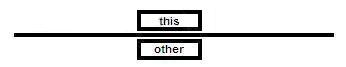Parameters:
`other` - another interval whose relation to this interval is to be investigated
Returns:
`true` if this interval is temporally equivalent to the other one else `false`
Since:
2.0
• ### precedes

public boolean precedes(I other)

ALLEN-relation: Does this interval precede the other one such that there is a gap between?

Relation diagram:Parameters:
`other` - another interval whose relation to this interval is to be investigated
Returns:
`true` if this interval is before the other such that there is a gap between else `false`
Since:
2.0
`precededBy(IsoInterval)`
• ### precededBy

public boolean precededBy(I other)

ALLEN-relation: Equivalent to `other.precedes(this)`.

Relation diagram: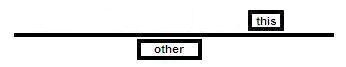Parameters:
`other` - another interval whose relation to this interval is to be investigated
Returns:
`true` if this interval is after the other such that there is a gap between else `false`
Since:
2.0
`precedes(IsoInterval)`
• ### meets

public boolean meets(I other)

ALLEN-relation: Does this interval precede the other one such that there is no gap between?

Relation diagram: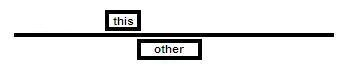Parameters:
`other` - another interval whose relation to this interval is to be investigated
Returns:
`true` if this interval is before the other such that there is no gap between else `false`
Since:
2.0
`metBy(IsoInterval)`
• ### metBy

public boolean metBy(I other)

ALLEN-relation: Equivalent to `other.meets(this)`.

Relation diagram: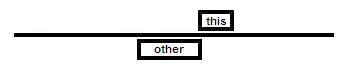Parameters:
`other` - another interval whose relation to this interval is to be investigated
Returns:
`true` if this interval is after the other such that there is no gap between else `false`
Since:
2.0
`meets(IsoInterval)`
• ### overlaps

public boolean overlaps(I other)

ALLEN-relation: Does this interval overlaps the other one such that the start of this interval is still before the start of the other one?

Relation diagram: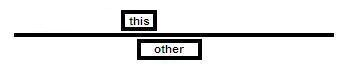Parameters:
`other` - another interval whose relation to this interval is to be investigated
Returns:
`true` if this interval overlaps the other such that the start of this interval is still before the start of the other one else `false`
Since:
2.0
`overlappedBy(IsoInterval)`, `intersects(ChronoInterval)`
• ### overlappedBy

public boolean overlappedBy(I other)

ALLEN-relation: Equivalent to `other.overlaps(this)`.

Relation diagram:Parameters:
`other` - another interval whose relation to this interval is to be investigated
Returns:
`true` if the other interval overlaps this such that the start of the other one is still before the start of this interval else `false`
Since:
2.0
`overlaps(IsoInterval)`, `intersects(ChronoInterval)`
• ### finishes

public boolean finishes(I other)

ALLEN-relation: Does this interval finish the other one such that both end time points are equal and the start of this interval is after the start of the other one?

Relation diagram: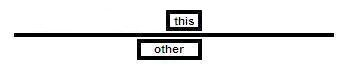Parameters:
`other` - another interval whose relation to this interval is to be investigated
Returns:
`true` if this interval has the same end point as the other one and a later start else `false`
Since:
2.0
`finishedBy(IsoInterval)`
• ### finishedBy

public boolean finishedBy(I other)

ALLEN-relation: Equivalent to `other.finishes(this)`.

Relation diagram: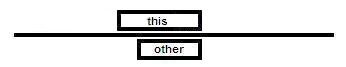Parameters:
`other` - another interval whose relation to this interval is to be investigated
Returns:
`true` if this interval has the same end point as the other one and an earlier start else `false`
Since:
2.0
`finishes(IsoInterval)`
• ### starts

public boolean starts(I other)

ALLEN-relation: Does this interval start the other one such that both start time points are equal and the end of this interval is before the end of the other one?

Relation diagram: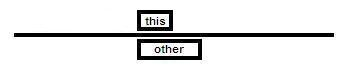Parameters:
`other` - another interval whose relation to this interval is to be investigated
Returns:
`true` if this interval has the same start point as the other one and an earlier end else `false`
Since:
2.0
`startedBy(IsoInterval)`
• ### startedBy

public boolean startedBy(I other)

ALLEN-relation: Equivalent to `other.starts(this)`.

Relation diagram: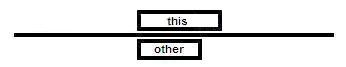Parameters:
`other` - another interval whose relation to this interval is to be investigated
Returns:
`true` if this interval has the same start point as the other one and a later end else `false`
Since:
2.0
`starts(IsoInterval)`
• ### encloses

public boolean encloses(I other)

ALLEN-relation: Does this interval enclose the other one such that this start is before the start of the other one and this end is after the end of the other one?

Relation diagram: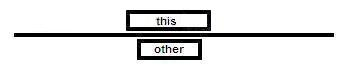Parameters:
`other` - another interval whose relation to this interval is to be investigated
Returns:
`true` if this interval has the earlier start point and later end compared to the other one else `false`
Since:
2.0
`enclosedBy(IsoInterval)`
• ### enclosedBy

public boolean enclosedBy(I other)

ALLEN-relation: Equivalent to `other.encloses(this)`.

Relation diagram: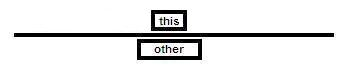Parameters:
`other` - another interval whose relation to this interval is to be investigated
Returns:
`true` if this interval has the later start point and earlier end compared to the other one else `false`
Since:
2.0
`encloses(IsoInterval)`
• ### intersects

public boolean intersects(ChronoInterval<T> other)

Queries if this interval intersects the other one such that there is at least one common time point.

Specified by:
`intersects` in interface `ChronoInterval<T extends Temporal<? super T>>`
Parameters:
`other` - another interval which might have an intersection with this interval
Returns:
`true` if there is an non-empty intersection of this interval and the other one else `false`
Since:
3.19/4.15
`findIntersection(ChronoInterval)`, `overlaps(IsoInterval)`, `isBefore(ChronoInterval)`, `isAfter(ChronoInterval)`
• ### findIntersection

public  findIntersection(ChronoInterval<T> other)

Obtains the intersection of this interval and other one if present.

Parameters:
`other` - another interval which might have an intersection with this interval
Returns:
a wrapper around the found intersection or an empty wrapper
Since:
4.21
`Optional.isPresent()`, `intersects(ChronoInterval)`
• ### abuts

public boolean abuts(ChronoInterval<T> other)

Queries if this interval abuts the other one such that there is neither any overlap nor any gap between.

Equivalent to the expression `this.meets(other) || this.metBy(other)`. Empty intervals never abut.

Specified by:
`abuts` in interface `ChronoInterval<T extends Temporal<? super T>>`
Parameters:
`other` - another interval which might abut this interval
Returns:
`true` if there is no intersection and no gap between else `false`
Since:
3.19/4.15
`meets(IsoInterval)`, `metBy(IsoInterval)`
• ### withValue

public <V> ValueInterval<T,​I,​V> withValue(V value)

Creates a combination of this interval with an associated value.

Type Parameters:
`V` - generic value type
Parameters:
`value` - associated value, not `null`
Returns:
new value interval
Since:
3.31/4.26